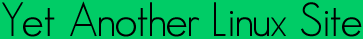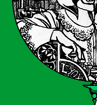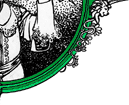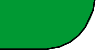Quick ?s
Cheat Sheets
Man Pages
The Lynx
Software
 ```J0(3) Linux Programmers Manual J0(3) NAME j0, j0f, j0l, j1, j1f, j1l, jn, jnf, jnl, y0, y0f, y0l, y1, y1f, y1l, yn, ynf, ynl - Bessel functions SYNOPSIS #include double j0(double x); double j1(double x); double jn(int n, double x); double y0(double x); double y1(double x); double yn(int n, double x); float j0f(float x); float j1f(float x); float jnf(int n, float x); float y0f(float x); float y1f(float x); float ynf(int n, float x); long double j0l(long double x); long double j1l(long double x); long double jnl(int n, long double x); long double y0l(long double x); long double y1l(long double x); long double ynl(int n, long double x); Link with -lm. Feature Test Macro Requirements for glibc (see feature_test_macros(7)): For all of these functions: _SVID_SOURCE || _BSD_SOURCE || _XOPEN_SOURCE DESCRIPTION The j0() and j1() functions return Bessel functions of x of the first kind of orders 0 and 1, respectively. The jn() function returns the Bessel function of x of the first kind of order n. The y0() and y1() functions return Bessel functions of x of the second kind of orders 0 and 1, respectively. The yn() function returns the Bessel function of x of the second kind of order n. For the functions y0(), y1() and yn(), the value of x must be positive. For negative values of x, these functions return -HUGE_VAL. The j0f() etc. and j0l() etc. functions are versions that take and return float and long double values, respectively. CONFORMING TO The functions returning double conform to SVr4, 4.3BSD, POSIX.1-2001. BUGS There are errors of up to 2e-16 in the values returned by j0(), j1() and jn() for values of x between -8 and 8. COLOPHON This page is part of release 3.05 of the Linux man-pages project. A description of the project, and information about reporting bugs, can be found at http://www.kernel.org/doc/man-pages/. 2007-07-26 J0(3) ```Yals.net is © 1999-2009 Crescendo Communications Sharing tech info on the web for more than a decade! This page was generated Thu Apr 30 17:05:27 2009